##### Actions

Liouville formula

A relation that connects the Wronskian of a system of solutions and the coefficients of an ordinary linear differential equation.

Letbe an arbitrary system ofsolutions of a homogeneous system oflinear first-order equations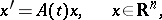(1)

with an operator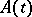that is continuous on an interval, and let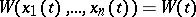be the Wronskian of this system of solutions. The Liouville–Ostrogradski formula has the form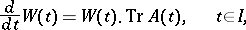(2)

or, equivalently,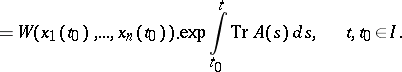Here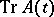is the trace of the operator. The Liouville–Ostrogradski formula can be written by means of the Cauchy operator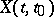of the system (1) as follows: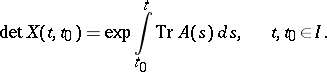(4)

The geometrical meaning of (4) (or ) is that as a result of the transformation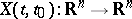the oriented volume of any body is increased by a factor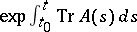.

If one considers a linear homogeneous-th order equation(5)

with continuous coefficients on an interval, and iffor, then the Liouville–Ostrogradski formula is the equality(6)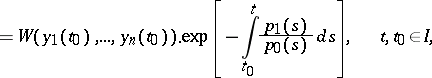where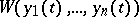is the Wronskian of the system ofsolutionsof (5). The Liouville–Ostrogradski formulas , (6) are ordinarily used in the case when the system of solutions in question is fundamental (cf. Fundamental system of solutions). For example, formula (6) makes it possible to find by quadratures the general solution of a linear homogeneous equation of the second order if one knows one particular non-trivial solution of it.

The relation (6) for equation (5) withwas found by N.H. Abel in 1827 (see ), and for arbitraryin 1838 by J. Liouville  and M.V. Ostrogradski ; the equality

was obtained by Liouville  and C.G.J. Jacobi  (as a consequence of this,

is sometimes called the Jacobi formula).

The Liouville–Ostrogradski formula (2) can be generalized to a non-linear system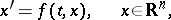(7)

under the assumption that the vector-valued function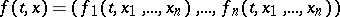and the matrix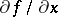are continuous. Ifis a set of finite measure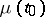and the imageof this set under the linear mapping, whereis the Cauchy operator of the system (7), has measure, then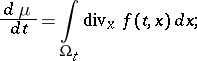here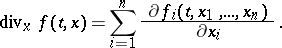This implies Liouville's theorem on the conservation of phase volume, which has important applications in the theory of dynamical systems and in statistical mechanics, mathematical problems in: The flow of a smooth autonomous systemdoes not change the volume of any body in the phase spaceif and only if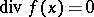for all; in particular, the phase volume is conserved by the flow of a Hamiltonian system.

How to Cite This Entry: